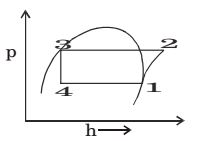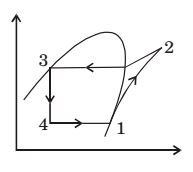## Refrigeration and Air-conditioning Miscellaneous

#### Refrigeration and Air-conditioning

1. Refrigerant vapor enters into the compressor of a standard vapor compression cycle at –10°C (h = 402 J/kg) and leaves the compression at 50°C (h = 432 kJ/kg). It leaves the condenser at 30°C (h = 237 kJ/kg). The COP of the cycle is ________.

1. Work done = 432 – 402 = 30 kJ/kg
Refrigerating effect = 402 – 237 = 165 kJ/kg.

 COP = 165 = 5.5 30

##### Correct Option: B

Work done = 432 – 402 = 30 kJ/kg
Refrigerating effect = 402 – 237 = 165 kJ/kg.

 COP = 165 = 5.5 30

1. The power required for compressor in kW is

1. Power required by compressor p = ṁ(h2 – h1)
= 0.2 (276.45 – 237) = 7.9 kW

##### Correct Option: C

Power required by compressor p = ṁ(h2 – h1)
= 0.2 (276.45 – 237) = 7.9 kW

1. The rate at which heat is extracted, in kJ/s from the refrigerated space is

1. Rate at which heat is extracted = ṁ(h1 – h4)
= 0.2 (237 – 95.5) = 28.3 kW

##### Correct Option: A

Rate at which heat is extracted = ṁ(h1 – h4)
= 0.2 (237 – 95.5) = 28.3 kW

1. In an ideal vapour compression refrigeration cycle, the specific enthalpy of refrigerant (in kJ/kg) at the following states is given as :
Inlet of condenser : 283
Exit of condenser : 116
Exit of evaporator : 232
The COP of this cycle is

1.1– 2 → work done by the compressor
2 – 3 → condenser heat rejected at constant pressure
3 – 4 → throttling
4 – 1 → heat addition in evaporator
Given: h2 = 283 kJ/ kg
h3 = 116 kJ/kg = h4 (from ph curve)
h1 = 232 kJ/kg
We know

 C.O.P = desired effect = Cooling effect work input work done by compressor

 C.O.P = h1 – h4 = 232 - 116 = 2.27 h2 – h1 283 - 232

##### Correct Option: A1– 2 → work done by the compressor
2 – 3 → condenser heat rejected at constant pressure
3 – 4 → throttling
4 – 1 → heat addition in evaporator
Given: h2 = 283 kJ/ kg
h3 = 116 kJ/kg = h4 (from ph curve)
h1 = 232 kJ/kg
We know

 C.O.P = desired effect = Cooling effect work input work done by compressor

 C.O.P = h1 – h4 = 232 - 116 = 2.27 h2 – h1 283 - 232

1. The vapour compression refrigeration cycle is represented as shown in the figure below, with state 1 being the exit of the evaporator. The coordinate system used in this figure is1. T-h curve

T-h curve